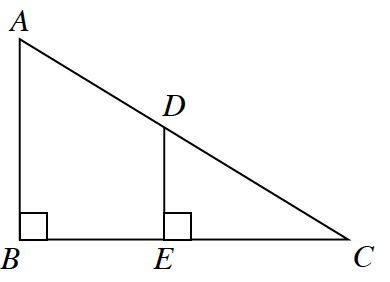### Home > INT2 > Chapter 2 > Lesson 2.3.2 > Problem2-96

2-96.

Are the triangles below similar? If so, write a flowchart proof that justifies your conclusion. If not, explain how you know.Draw triangles $ABC$ and $DEF$ separately. What do you notice about angles $B$ and $E$? What do you notice about angle $C$?